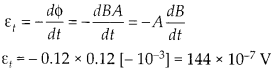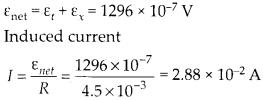Enlightened

# Question 12: NCERT Solutions for 12th Class Physics: Chapter 6-Electromagnetic Induction

• 0

Question 12: NCERT Solutions for 12th Class Physics: Chapter 6-Electromagnetic Induction

A square loop of side 12 cm with its sides ‘r parallel to X and Y axes is moved with a velocity of 8 cm s’1 in the positive x-direction in an environment containing a magnetic field in the positive z-direction. The field is neither uniform in space nor constant in time. It has a gradient of 10-3 T cm-1 along the negative x:direction (that is it increases by 10“3 T cm-1 as one moves in the negative x-direction) and it is decreasing in time at the rate of 10-3 T s–1. r Determine the direction and magnitude of the induced current in the loop if its resistance is 4.50 mΩ

Share

1. Solution:

Each side of square loop is 12 cm and magnetic field is decreasing along x direction.Also the magnetic field is decreasing with time at constant rateInduced emf and rate of change of magnetic flux due to only time variationInduced emf and rate of change of magnetic flux due to change in position.

Both the induced emf have same sign and thus adds to provide net Induced emf in the loopCheck the complete chapter with solutions.

NCERT Solutions for 12th Class Physics: Chapter 6-Electromagnetic Induction

• 0